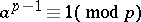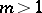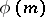# Fermat's little theorem

(diff) ← Older revision | Latest revision (diff) | Newer revision → (diff)
For a numbernot divisible by a prime number, the congruenceholds. This theorem was established by P. Fermat (1640). It proves that the order of every element of the multiplicative group of residue classes modulodivides the order of the group. Fermat's little theorem was generalized by L. Euler to the case modulo an arbitrary. Namely, he proved that for every numberrelatively prime to the given numberthere is the congruencewhereis the Euler function. Another generalization of Fermat's little theorem is the equation, which is valid for all elements of the finite fieldconsisting ofelements.